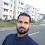### Section - I

1. Attempt any five parts of the following questios. Answers to each part should be of approximatel 50 words.

Marks: 7 × 5 = 35

#### (a) How do you draw a Lorenz curve? Explain its use.

(Comment for solution.)

#### (b) What is 'shadow price'? Why are shadow prices used in project analysis as against market prices?

(Comment for solution.)

#### (c) What is Engel's law? Which sector/product(s) of an economy operate under this law?

(Comment for solution.)

#### (d) State the first and second fundamental theorems of welfare economics, and comment on their usefulnesses.

(Comment for solution.)

#### (e) State the Kuhn - Tucker conditions.

(Comment for solution.)

#### (f) Explain how Pareto's law of distribution is useful in measuring income distribution.

(Comment for solution.)

#### (g) Explain total factor productivity and mention any two popular measures of the same.

(Comment for solution.)

### Section - II

Attempt any seven of the following questions. Each answer is to be in about 150 words.

Marks: 15 × 7 = 105

#### 2. Show how a demand function may be derived from the Cobb-Douglas utility function.

Solution (Only for IES Google Classroom Members)

#### 3. Formulate a translog cost function and show how the elasticity of input substitution may be obtained.

(Comment for solution.)

#### 4. What is "moral hazard" in economic theory? Discuss a situation that would describe a moral hazard problem.

(Comment for solution.)

#### 5. What is "free-rider" problem? Discuss the possible solutions ot this problem.

(Comment for solution.)

#### 6. State and explain the Coase theorem in the context of pollution control.

(Comment for solution.)

#### 7. Explain Leontief's static input-outut model and describe its limitations.

(Comment for solution.)

#### 8. What is "prisoner's dilemma"? How is it related to strictly dominant strategy?

Solution (Only for IES Google Classroom Members)

#### 9. What is a superlative index number? How is it related to the theory of aggregation?

(Comment for solution.)

#### 10. Define a quadratic form, and state the conditions under which it is (i) positive definite, (ii) positive sem-definite, and (iii) negative definite.

(Comment for solution.)

### Section - III

Attempt any two of the following questios, in about 500 each.

Marks: 30 × 2 = 60

#### 11. Explain the principl of least squares as a basis for multiple regression analysis. Also state the underlying standard assumptions of ordinary least Squares estimation. Explain further the consequences of violation of such assumptions.

(Comment for solution.)

#### 12. In the case of a pure exchange econom how do you characterize Walrasian equilibrium? Also establish the conditions under which such an equilibrium exists.

(Comment for solution.)

#### 13 (a). State and prove Euler's theorem. Is i relevant in the context of a firm?

(Comment for solution.)

#### 13 (b). Distinguish between technical and allocative efficiency in the context of a firm. Give an example.

(Comment for solution.)

#### 13 (c). Distinguish between parametric and non - parametric tests in testing of hypotheses.

(Comment for solution.)

1.Sir, when I substitute the demand function for good x in equation of budget constraint, i am unable to obtain the demand function of y. There is no Beta value while rearranging the equation..

2.But i got the correct demand function of Good Y when i substituted the value of demand function for good x in the equation where you found value of Y first and also used it in the budget constraint.

1.$x = \frac{{\alpha I}}{{{p_x}}}$
Substituting:
$I = {p_x} \times \frac{{\alpha I}}{{{p_x}}} + {p_y}y$
$I = \alpha I + {p_y}y$
${p_y}y = I - \alpha I$
$y = \frac{{I(1 - \alpha )}}{{{p_y}}}$
We know that:
$\alpha + \beta = 1$
$\beta = 1 - \alpha$
Therefore,
$y = \frac{{\beta I}}{{{p_y}}}$

3.Thank you sir